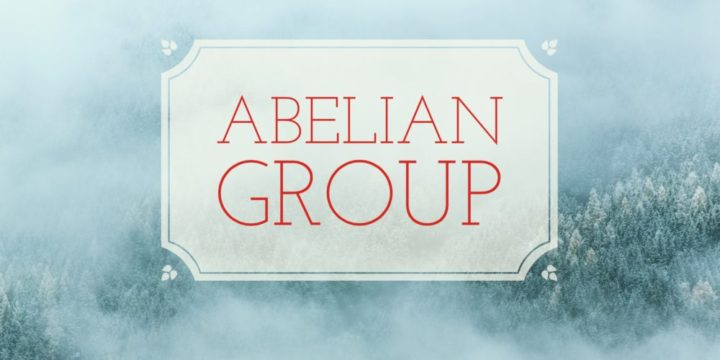# Abelian Normal Subgroup, Intersection, and Product of Groups## Problem 195

Let $G$ be a group and let $A$ be an abelian subgroup of $G$ with $A \triangleleft G$.
(That is, $A$ is a normal subgroup of $G$.)

If $B$ is any subgroup of $G$, then show that
$A \cap B \triangleleft AB.$Add to solve later

## Proof.

First of all, since $A \triangleleft G$, the product $AB$ is a subgroup of $G$.
To show that $A\cap B$ is a normal subgroup of $AB$, let $x\in A \cap B$ and $ab\in AB$, where $a\in A$ and $b \in B$.
Then we have the conjugate
\begin{align*}
(ab)x(ab)^{-1}=a(bxb^{-1})a^{-1}. \tag{*}
\end{align*}

We show that the right hand side of (*) is in both $A$ and $B$.
Since $x\in A\cap B \subset A$ and $A$ is a normal subgroup of $G$, we have
$bxb^{-1}\in A.$ Thus the right hand side of (*) is in $A$.

Also, since the elements $a, bxb^{-1}, a^{-1}$ are all in the abelian group $A$, we have
\begin{align*}
a(bxb^{-1})a^{-1}=aa^{-1}(bxb^{-1})=bxb^{-1}\in B
\end{align*}
since $x, b\in B$.

Therefore $(ab)x(ab)^{-1}$ is in both $A$ and $B$, and hence
$(ab)x(ab)^{-1} \in A \cap B$ and the group $A \cap B$ is a normal subgroup of $AB$.Add to solve later

### More from my site

#### You may also like...

This site uses Akismet to reduce spam. Learn how your comment data is processed.

###### More in Group Theory##### Abelian Groups and Surjective Group Homomorphism

Let $G, G'$ be groups. Suppose that we have a surjective group homomorphism $f:G\to G'$. Show that if $G$ is...

Close